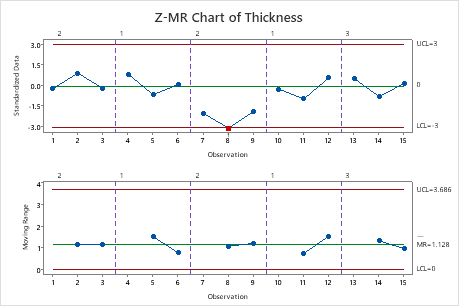# Interpret the key results for a Z-MR Chart

Complete the following steps to interpret a Z-MR chart. Key output includes the Z chart, the Moving Range chart, and test results.

## Step 1: Determine whether the process variation is stable and in control

The Moving Range chart plots the moving ranges. The center line is the average of all moving ranges. The control limits on the Moving Range chart, which are set at a distance of 3 standard deviations above and below the center line, show the amount of variation that is expected in the moving ranges of the standardized data..

Red points indicate observations that fail at least one of the tests for special causes and are not in control. If the same point fails multiple tests, then the point is labeled with the lowest test number to avoid cluttering the graph. If the chart shows out-of-control points, investigate those points.

Out-of-control points can influence the estimates of process parameters and prevent control limits from truly representing your process. If out-of-control points are due to special causes, then consider omitting these points from the calculations. For more information, go to Specify how to estimate the parameters for Z-MR Chart.In these results, no points are out of control on the Moving Range chart. The process variation is stable over time, so you can interpret the Z chart.

## Step 2: Determine whether the process mean is in control

The Z chart plots the standardized individual observations by subtracting the mean to center the data, then dividing by the standard deviation. The center line is the estimate of the process average across runs. The control limits, which are set at a distance of 3 standard deviations above and below the center line, show the amount of variation that is expected in the standardized observations.

Red points indicate observations that fail at least one of the tests for special causes and are not in control. If the same point fails multiple tests, then the point is labeled with the lowest test number to avoid cluttering the graph. If the chart shows out-of-control points, investigate those points.In these results, one point is out of control on the Z chart. The standardized value for observation 8 is below the lower control limit. The process center is not stable across runs. When you hold the pointer over a red point, you can get more information about this point.

## Step 3: Identify which points failed each test

Investigate any observations that failed the tests for special causes. By default, Minitab conducts only Test 1, which detects points that fall outside of the control limits. However, if you conduct additional tests, then points can fail multiple tests. The output shows exactly which points failed each test, as shown here.

### Test Results for Z Chart of Thickness

TEST 1. One point more than 3.00 standard deviations from center line. Test Failed at points: 8 * WARNING * If graph is updated with new data, the results above may no longer be correct.
###### Note

When you use several tests at the same time, the sensitivity of the chart increases. However, the false alarm rate also increases, which can make you react to the test results unnecessarily.

For more information on each of the tests and when to use them, go to Using tests for special causes in control charts.